SPSS TUTORIALS BASICS ANOVA REGRESSION FACTOR CORRELATION

# SPSS Datetime Variables Tutorial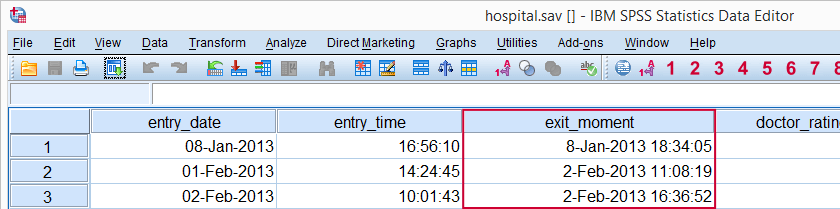## SPSS Main Datetime Functions

This tutorial will cover the datetime functions outlined in the table below. Most of them apply to SPSS date variables and time variables as well because their values are stored in numbers of seconds too.

FunctionUseExampleReturns
DATESUMAdd number of given time units to datetime variableDATESUM(datetime,1,'months')Time value
DATEDIFFCompute difference between two datetime variables in given time unitDATEDIFF(datetime1,datetime2,'hours')Standard numeric value
CTIMEConvert seconds to other time unit without truncationCTIME.DAYS(timespan)Standard numeric value
XDATEExtract date or time component from datetime variableXDATE.HOURS(time)Standard numeric value
DATE.DMYCreate date value from day, month and yearDATE.DMY(10,2,2015)Date value
TIME.HMSCreate time value from hours, minutes, secondsTIME.HMS(20,15,30)Time value

## SPSS Date and Time to Datetime

We'll first combine entry_date and entry_time into entry_moment because we'll need it a bit later on. The syntax below shows how to do so with a very basic COMPUTE command followed by FORMATS.

*1. Combine date and time into datetime (in seconds).

compute entry_moment = entry_date + entry_time.
exe.

*2. Display seconds as normal date with time.

formats entry_moment(datetime20).

## SPSS DATESUM Function

SPSS DATESUM adds to datetime variables a given number of time units (days, hours and so on). Specify a negative number of time units for subtraction. For example, the hospital staff wants to contact their patients for a survey exactly 1 month after they've left the hospital. A notification should be sent 7 days prior to contacting patients.
Both datetime variables are easily created with SPSS DATESUM as shown in the syntax below. The result in data view is shown in the following screenshot.

*1. Compute contact_date = 1 month after leaving hospital.

compute contact_date = datesum(exit_moment,1,'months').
exe.

*2. Show contact_date as normal dates with times.

formats contact_date(datetime20).

*3. Compute notify_date as 7 days prior to contact_date.

compute notify_date = datesum(contact_date,-7,'days').
exe.

*4. Show notify_date as normal dates with times.

formats notify_date (datetime20).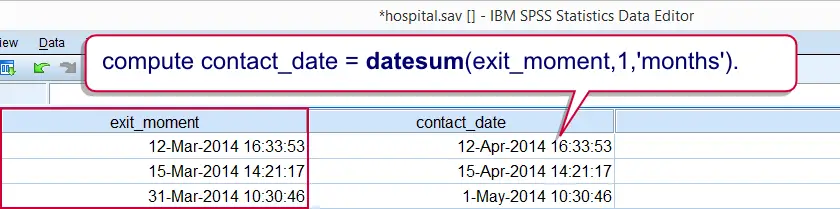## SPSS DATEDIFF Function

SPSS DATEDIFF function returns the difference between two datetime values in a given time unit (hours, days and so on). For example, how long did the patients stay in the hospital? The syntax below first answers the question by using DATEDIFF.
Now, keep in mind that DATEDIFF truncates (rounds down) its return values. Personally, we prefer using a basic subtraction here. Because datetime values are numbers of seconds, the result is a number of seconds too. However, we can easily show these as hours, minutes and seconds by giving it a time format. This is shown in the second example below.

## SPSS DATEDIFF Syntax Example

*1. Compute duration in days with DATEDIFF.

compute duration_days = datediff(exit_moment,entry_moment,'days').
exe.

*2. Compute duration in seconds by basic subtraction.

compute duration_time = exit_moment - entry_moment.
exe.

*3. Show duration_time in hours, minutes, seconds.

formats duration_time(time8).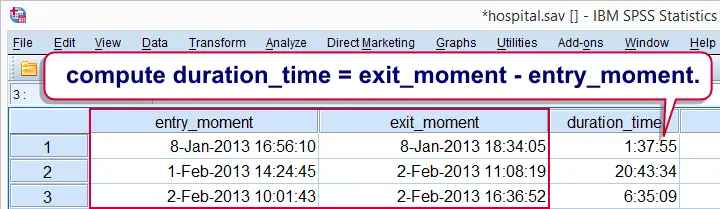## SPSS CTIME Function

SPSS CTIME converts seconds to other time units such as hours, days or months.Oddly, CTIME.YEARS is painfully missing in SPSS while CTIME.SECONDS -which does basically nothing- is present instead. In contrast to DATEDIFF, return values aren't truncated.
Note that duration_time is a time variable so it really holds numbers of seconds. We can convert those to the desired time units with CTIME as demonstrated below; the syntax recalculates duration in days but this time without truncating the outcome values.
An alternative to CTIME here is using a basic division; since a day holds 86,400 seconds, dividing time values by 86400 is equivalent to using CTIME.DAYS. This is shown in the second example below.

## SPSS CTIME Syntax Example

*1. Convert duration_time (seconds) to duration_day.

compute duration_days = ctime.days(duration_time).
exe.

*2. Alternative second to day conversion.

compute duration_days = duration_time / 86400.
exe.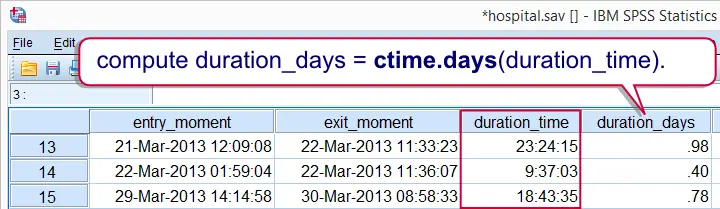## SPSS XDATE Function

SPSS XDATE extracts date components from date, time and datetime values. The syntax below thus shows how to extract the day, month and year from exit_moment. Note that XDATE.MDAY rather than XDATE.DAY returns the day of the month.

*Extract day, month and year from datetime with XDATE.

compute exit_day = xdate.mday(exit_moment).
compute exit_month = xdate.month(exit_moment).
compute exit_year = xdate.year(exit_moment).
exe.

## SPSS Datetime Comparisons

Comparing two SPSS datetime variables is straightforward and can be done with the usual operators such as >, <= and others. For example, exit_moment must obviously be greater (later in time) than entry_moment for all visits. The syntax confirms that this holds for all visits by using IF.

## SPSS Datetime Comparison Example 1

*1. Compute new variable holding just zeroes.

compute exit_after_entry = 0.

*2. Compare datetimes to detect abnormal cases.

if exit_moment > entry_moment exit_after_entry = 1.

*3. All cases flagged, no abnormalities.

frequencies exit_after_entry.

As we mentioned before, datetime values are numbers of seconds. You can compare datetime values to a given date by converting the latter into seconds as well. This is readily done by DATE.DMY as we'll show in a minute.
For example, a report criticizing the hospital was published on November 2nd., 2014. As a first step in evaluating its impact on patient ratings, we'll flag all visits that ended on or after this date.The COMPUTE command used in the syntax below looks a bit weird. It is explained in Compute A = B = C. Keep in mind that date values are identical to datetime values with 00:00:00 as their time components.

## SPSS Datetime Comparison Example 2

*Flag all cases whose exit_moment is during or after November 2nd., 2014.

compute after_report = exit_moment > date.dmy(2,11,2014).
exe.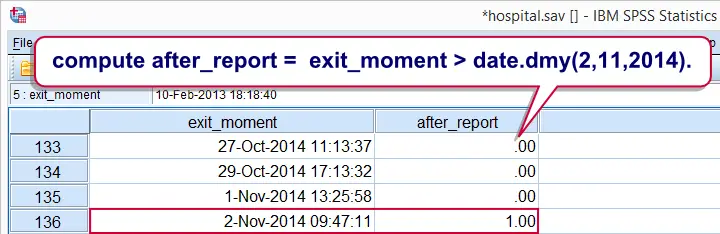The report was published at 12:10:05 (10 minutes, 5 seconds past noon). Note that two visits ending on the publication date but before the publication time were flagged. How can we exclude such cases?
Well, remember that an SPSS datetime value is identical to the sum of an SPSS date value and an SPSS time value. The syntax below uses this in order to compare a datetime variable to a given date and time.

## SPSS Datetime Comparison Example 3

*Flag cases whose exit was later than November 2nd., 12:10:05 in the afternoon.

compute after_report = exit_moment > date.dmy(2,11,2014) + time.hms(12,10,5).
exe.

# Tell us what you think!

*Required field. Your comment will show up after approval from a moderator.

# THIS TUTORIAL HAS 2 COMMENTS:

•### By PIERRE GARRAUD on October 15th, 2015

Let me try to make myseft understood:
If i want to assign a numeric value to variable, i use this syntax : if (index=1) var=12.
a character variable, if (index=1) var="12".
how can i do it for datetime variable ?

Pierre
thanks a lot

•### By Ruben Geert van den Berg on October 16th, 2015

`*Create data example.`
``` data list free/id. begin data 1 2 3 4 5 end data. *Insert October 13 2015, 9:08:17 AM for cases whose \$casenum > 3. if (\$casenum > 3) now = date.dmy(13,10,2015) + time.hms(9,8,17). execute. *Results in huge number of seconds between 1582 and desired datetime. Display seconds as normal datetime. ```
`formats now (datetime22).`